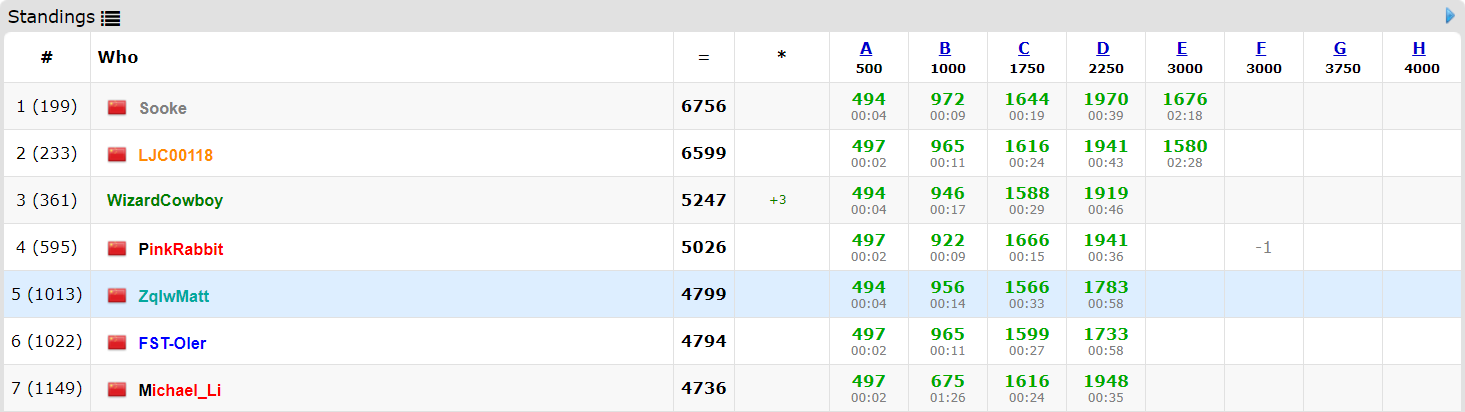# ZqlwMatt

• ### 「OI」比赛赛后记录贴

• ##### 2018-05-05：「JSOI2018」 R3.4 划水记
• ...... 立个flag，接下来努力记录每一场比赛模拟赛除外

### 2018-10-14：Codeforces Round #516 (Div. 2, by Moscow Team Olympiad)

• emm...一场在机房开黑打的CF
• Writers 超好看！好评     群里爆D壮观FST，紧张刺激qwq...，于是围观D！最后rk121 -> rk46 提升75 （FSTforces

C. Oh Those Palindromes 简要题解：（比赛时后面有人喊了句sort后输出就好了
正确性证明：也就是每个字符c出现了x次，那么作为回文串的端点至多被匹配 次。 而构造连续恰能满足上界。

E. Dwarves, Hats and Extrasensory Abilities 简要题解：
题中的 $n$ 非常小，我们考虑将所有黑点和白点构造在一条直线的两边（不妨全放在x轴上）
假设你已经有了一堆黑点在x轴左方和一堆白点在x轴右方，你会怎么做？
很容易想到取左方最右边界和右方最左边界，在它们之间再询问一个点，是哪种颜色就归到那一边。
最后只要给出两边界之间的直线就行了。全过程可以二分实现。不难.

### 2018-11-24：Codeforces Round #524 (Div. 2)

• 这次嘛，又双叒叕是跟着AK NOIP的LiM大佬打的 Codeforces Round。

C. Masha and two friends 矩形求交的套路题。可以确定 $(a_1,b_1,a_2,b_2)$$(c_1,d_1,c_2,d_2)$ 交完的矩形是 $\{\max(a_1,c_1),\max(b_1,d_1),min(a_2,c_2),min(b_2,d_2)\}$

D. Olya and magical square RE的程序在Codeforces上变成WA，自闭了......

考虑贪心，先尽可能小地分答案，然后发现划成 $2^{n-x}$ 次的最小代价是 $2^{x+1}-x-2$ ，然后再在非路径的格子里尽量划分。我们用 $f(x)$ 表示边长为 $2^{x}$ 的正方形能划多少刀，于是有 $f(x)=4 \times f(x-1)+1$ 然后最多只有 $log_{4}(10^{18})<32$ 所以 $n \ge 32$ 可以直接判掉。
对于 $n < 32$ ，观察到剩余格子里边长为 $n-i~(i=1,2,...)$ 的数量为 $1,5,13,31...$ 即满足 $num(i)=num(i-1) \times 2 + 3$ 。然后判断 $k$ 是否过大就好了。

### 2018-12-23：Codeforces Round #528 (Div. 1, based on Technocup 2019 Elimination Round 4)

旁观Div.2，秒了A，B，C，D (Div.2) 开始打隔膜C. Vasya and Templates 题解：贪心。
对于 $s$ 从左到右，尽可能小地确定最高位的字符。如 $s$=bbcb$a$=aada，就将 b $\rightarrow$ a。此时 $s$=aaca，发现 c $\le$ d ，将 c $\rightarrow$ d。若匹配到最后发现初始确定的最高位的字符过小的话，就将其值 $+1$ 重新匹配，那么此时已经有 $s \ge a$ 。继续向后贪心即可。时间复杂度：$\mathcal{O(n \times k^{2})}$

### 2018-12-30：Good Bye 2018全场出四题系列。。。